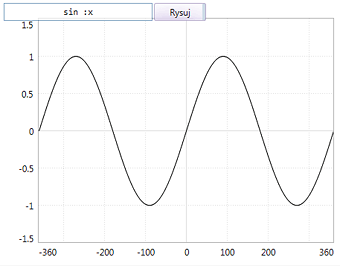## y = f(x)Enter any function formula in the textbox, using POOL syntax for the mathematical expressions. Use `:x` variable as the function's argument, e.g.: `2 * :x + 1` or `log :x^2`.

Program compiles and runs the code from textbox for `:x` in the range adjusted to the current drawing scale. Change of the drawing scale or resize/scroll of the window raises the "worldchanged" event. Handling function for this event redraws the plot.
Turn on the coordinate system in POOL options. You can change there also x and y scales and the center shift. Such changes will cause the automatic redraw.

The function value is calculated with the following expression: `"y := run :fn`, where `:fn` is the list that contains the function formula. This allows for execution of formulas more complex than a single equation. Try to enter in the textbox e.g.: `let "r (runif 0 1) :r^2`.